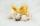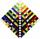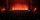# Octahedron - sum

On each wall of a regular octahedron is written one of the numbers 1, 2, 3, 4, 5, 6, 7 and 8, wherein on different sides are different numbers. For each wall John make the sum of the numbers written of three adjacent walls. Thus got eight sums, which also added up.
What values the resulting sum may have?

Result

s =  144

#### Solution:Leave us a comment of example and its solution (i.e. if it is still somewhat unclear...):

Showing 0 comments:Be the first to comment!## Next similar examples:

1. TunnelsMice had built an underground house consisting of chambers and tunnels: • each tunnel leading from the chamber to the chamber (none is blind) • from each chamber lead just three tunnels into three distinct chambers, • from each chamber mice can get to any
2. SeatsSeats in the sport hall are organized so that each subsequent row has five more seats. First has 10 seats. How many seats are: a) in the eighth row b) in the eighteenth row
3. Consecutive numbersSum of ten consecutive numbers is 105. Determine these numbers (write first and last).
4. Basket of fruitIn six baskets, the seller has fruit. In individual baskets, there are only apples or just pears with the following number of fruits: 5,6,12,14,23 and 29. "If I sell this basket," the salesman thinks, "then I will have just as many apples as a pear." Which
5. SequenceBetween numbers 1 and 53 insert n members of the arithmetic sequence that its sum is 702.
6. TreesA certain species of tree grows an average of 0.5 cm per week. Write an equation for the sequence that represents the weekly height of this tree in centimeters if the measurements begin when the tree is 200 centimeters tall.
7. Sequence 2Write the first 5 members of an arithmetic sequence a11=-14, d=-1
8. Sequence 3Write the first 5 members of an arithmetic sequence: a4=-35, a11=-105.
9. SequenceWrite the first 6 members of these sequence: a1 = 5 a2 = 7 an+2 = an+1 +2 an
10. SequenceWrite the first 7 members of an arithmetic sequence: a1=-3, d=6.
11. Theorem proveWe want to prove the sentense: If the natural number n is divisible by six, then n is divisible by three. From what assumption we started?
12. AS sequenceIn an arithmetic sequence is given the difference d = -3 and a71 = 455. a) Determine the value of a62 b) Determine the sum of 71 members.
13. AP - simpleDetermine the first nine elements of sequence if a10 = -1 and d = 4
14. Nineteenth memberFind the nineteenth member of the arithmetic sequence: a1=33 d=5 find a19
15. SeriesYour task is express the sum of the following arithmetic series for n = 14: S(n) = 11 + 13 + 15 + 17 + ... + 2n+9 + 2n+11
16. StudentsIn the front row sitting three students and in every other row 11 students more than the previous row. Determine how many students are in the room when the room is 9 lines, and determine how many students are in the seventh row.
17. 6 termsFind the first six terms of the sequence. a1 = 7, an = an-1 + 6